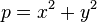You are currently browsing the tag archive for the ‘fermat’ tag.Fermat’s Theorem on the sum of two squares

Not as famous as Fermat’s Last Theorem (which baffled mathematicians for centuries), Fermat’s Theorem on the sum of two squares is another of the French mathematician’s theorems.

Fermat asserted that all odd prime numbers p of the form 4n + 1 can be expressed as:where x and y are both integers.  No prime numbers of the form 4n+3 can be expressed this way.

This is quite a surprising theorem – why would we expect only some prime numbers to be expressed as the sum of 2 squares?  To give some examples:

13 is a prime number of the form 4n+1 and can be written as 32 + 22.
17 is also of the form 4n + 1 and can be written as 42 + 12.
29 = 52 + 22.
37 = 62 + 12.

Prime numbers of the form 4n + 3 such as 7, 11, 19 can’t be written in this way.

The proof of this theorem is a little difficult.  It is however easier to prove a similar (though not logically equivalent!) theorem:

All sums of x2 + y2 (x and y integers) are either of the form 4n + 1 or even.

In other words, for some n:

x2 + y2 = 4n + 1 or

x2 + y2 = 2n

We can prove this by looking at the possible scenarios for the choices of x and y.

Case 1:

x and y are both even (i.e. x = 2n and y = 2m for some n and m).  Then

x2 + y2 = (2n)2 + (2m)2
x2 + y2 = 4n2 + 4m2
x2 + y2 = 2(2n2 + 2m2)

which is even.

Case 2:

x and y are both odd (i.e. x = 2n+1 and y = 2m+1 for some n and m).
Then x2 + y2 = (2n+1)2 + (2m+1)2
x2 + y2 = 4n2+ 4n + 1 + 4m2 + 4m + 1
x2 + y2 = 4n2+ 4n + 4m2 + 4m + 2
x2 + y2 = 2(2n2 + 2m2 + 2m + 2n + 1).
which is even.

Case 3:

One of x and y is odd, one is even. Let’s say x is odd and y is even. (i.e. x = 2n+1 and y = 2m for some n and m).
Then x2 + y2 = (2n+1)2 + (2m)2
x2 + y2 = 4n2+ 4n + 1 + 4m2
x2 + y2 = 4(n2+m2+n) + 1
which is in the form 4k+1 (with k = (n2+m2+n) )

Therefore, the sum of any 2 integer squares will either be even or of the form 4n+1. Unfortunately this does not necessarily imply the reverse: that all numbers of the form 4n+1 are the sum of 2 squares (which would then prove Fermat’s Theorem). This is because,

A implies B
Does not necessarily mean that
B implies A

For example,

If A is “cats” and B is “have 4 legs”
A implies B (All cats have 4 legs)
B implies A (All things with 4 legs are cats).

A is logically sound, whereas B is clearly false.

This is a nice example of some basic number theory – such investigations into expressing numbers as the composition of 2 other numbers have led to some of the most enduring and famous mathematical puzzles.

The Goldbach Conjecture  suggests that every even number greater than 2 can be expressed as the sum of 2 primes and has remained unsolved for over 250 years. Fermat’s Last Theorem lasted over 350 years before finally someone proved that an + bn=c2 has no positive integers a, b, and c which solve the equation for n greater than 2.

If you liked this post you might also like:

The Goldbach Conjecture – The Goldbach Conjecture states that every even integer greater than 2 can be expressed as the sum of 2 primes.  No one has ever managed to prove this.

Mathematical Proof and Paradox – how we can “prove” the impossible

Essential resources for IB students:I’ve put together four comprehensive pdf guides to help students prepare for their exploration coursework and Paper 3 investigations. The exploration guides talk through the marking criteria, common student mistakes, excellent ideas for explorations, technology advice, modeling methods and a variety of statistical techniques with detailed explanations. I’ve also made 17 full investigation questions which are also excellent starting points for explorations.  The Exploration Guides can be downloaded here and the Paper 3 Questions can be downloaded here.

### Website Stats

• 9,478,123 views

All content on this site has been written by Andrew Chambers (MSc. Mathematics, IB Mathematics Examiner).

### New website for International teachersI’ve just launched a brand new maths site for international schools – over 2000 pdf pages of resources to support IB teachers.  If you are an IB teacher this could save you 200+ hours of preparation time.

Explore here!

### Free HL Paper 3 QuestionsP3 investigation questions and fully typed mark scheme.  Packs for both Applications students and Analysis students.A Super Exploration Guide with 168 pages of essential advice from a current IB examiner to ensure you get great marks on your coursework.